SYLLABUS  Previous: 2.5 Implicit Crank-Nicholson  Up: 2.5 Implicit Crank-Nicholson  Next: 2.5.2 Schrödinger equation

### 2.5.1 Advection-diffusion equation

With Crank-Nicholson, the centered differences in space are evaluated in the same manner and with equal weights for the present and the future values of the function: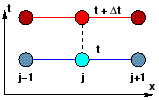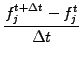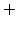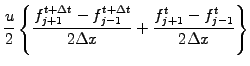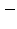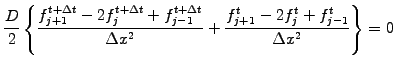The scheme can conveniently be recast into a linear system with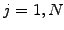equations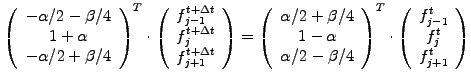(2.5.1#eq.11)

where the band matrix on the left has three diagonals and the right hand side can be evaluated explicitly from already known quantities. Performing a von Neumann stability analysis for a pure diffusive process (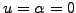) yields the amplification factor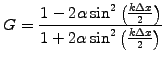(2.5.1#eq.12)

This shows that the Crank-Nicholson scheme is unconditionally stable (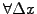,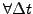) with phase errors affecting mainly short wavelengths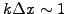. A similar conclusion can also be reached for the advective part. The Crank-Nicholson scheme (2.5.1#eq.12) has been implemented in JBONE with
      BandMatrix a = new BandMatrix(3, f.length);
BandMatrix b = new BandMatrix(3, f.length);
double[]   c = new double[f.length];
for (int j=0; j<=n; j++) {
a.setL(j,-0.25*beta -0.5*alpha);             //Matrix elements
a.setD(j,            1. +alpha);
a.setR(j, 0.25*beta -0.5*alpha);
b.setL(j, 0.25*beta +0.5*alpha);             //Right hand side
b.setD(j,            1. -alpha);
b.setR(j,-0.25*beta +0.5*alpha);
}
c=b.dot(f);                                    //Right hand side
c=c+b.getL(0)*f[n];                      // with periodicity
c[n]=c[n]+b.getR(n)*f;

fp=a.solve3(c);                                //Solve linear problem

The BandMatrix.solve3() method solves the linear system efficiently in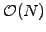operations, using an LU-factorization that will be discussed later in sect.3.

The favorable stability property (2.5.1#eq.13) can nicely be exploited in diffusion dominated problems dealing with the evolution of large scale features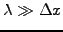. Starting from a relatively smooth Gaussian pulse that is subject to both advection and diffusion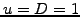, the applet below shows that a reasonably accurate solution (12 % for the valley to peak ratio when the time reaches 100) can be computed using extremely large time steps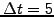, where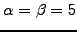.

 Numerical experiments: Crank-Nicholson for advection-diffusion Switch to an initial Box function; explain what happens after one time step. Complete the Implicit 2-level calculation with a Box and terminate with a few tiny TimeStep=0.1 using the Explicit 2-level scheme (2.1#eq.1). Compare the result with the best solution you have obtained so far.

SYLLABUS  Previous: 2.5 Implicit Crank-Nicholson  Up: 2.5 Implicit Crank-Nicholson  Next: 2.5.2 Schrödinger equation